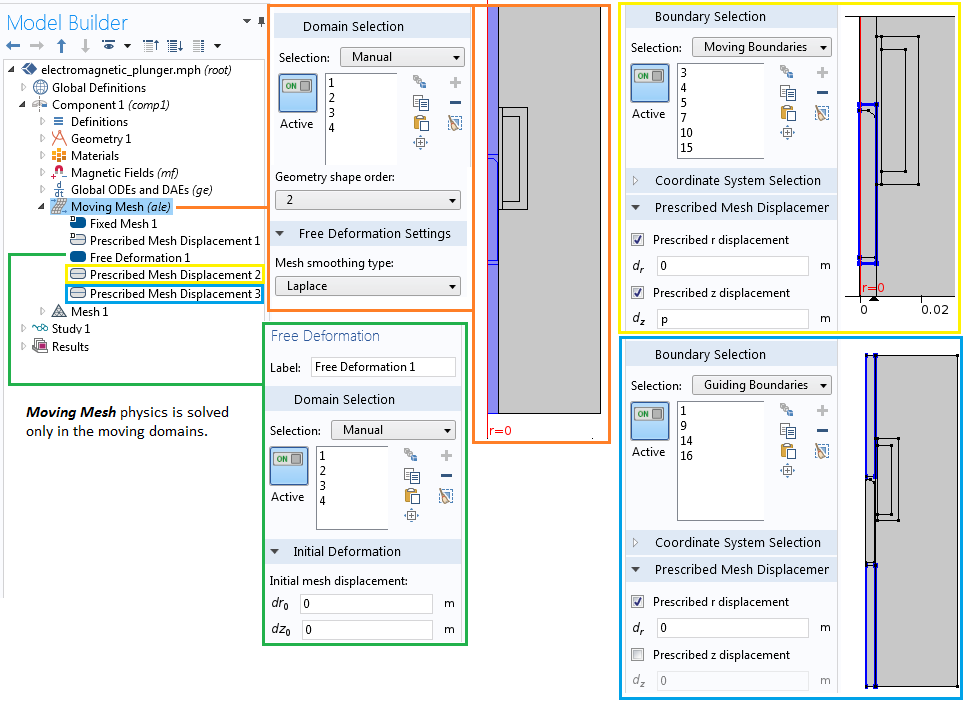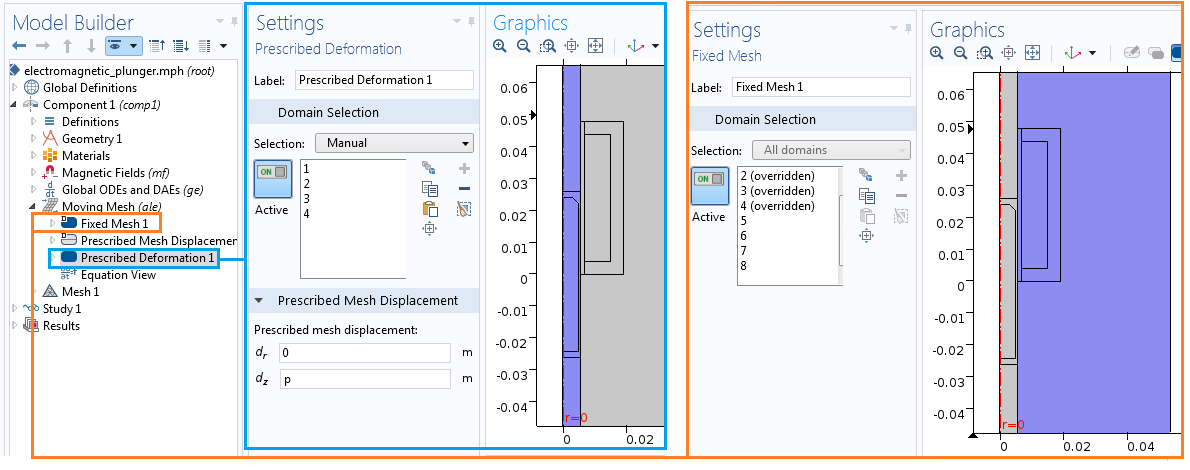第一部分：如何模拟线性电磁柱塞

电磁执行器的广泛工业应用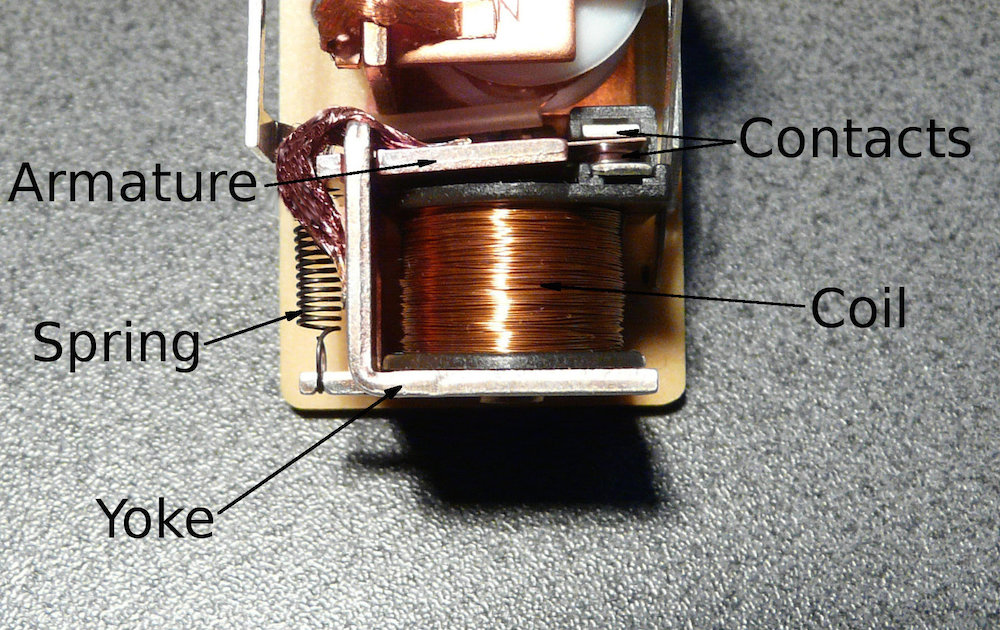在 COMSOL Multiphysics 中模拟线性电磁柱塞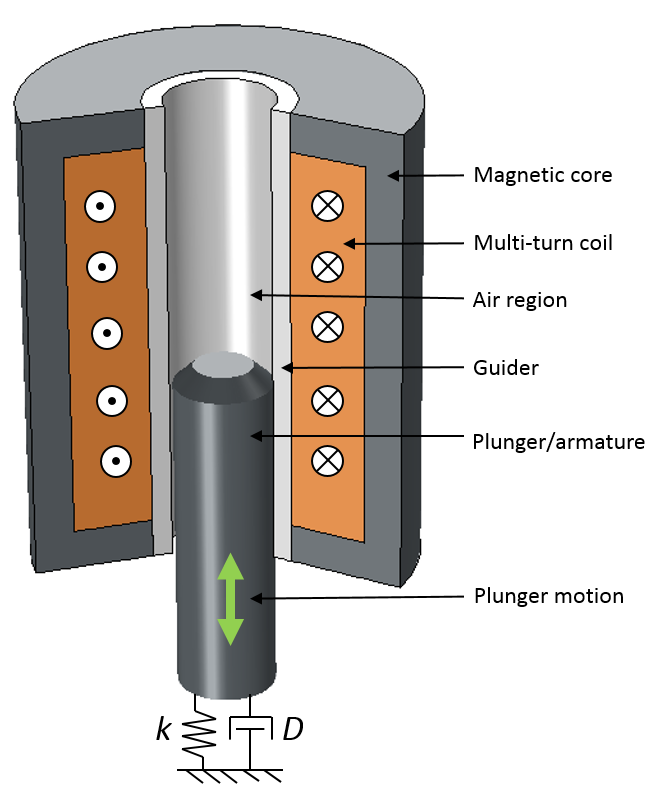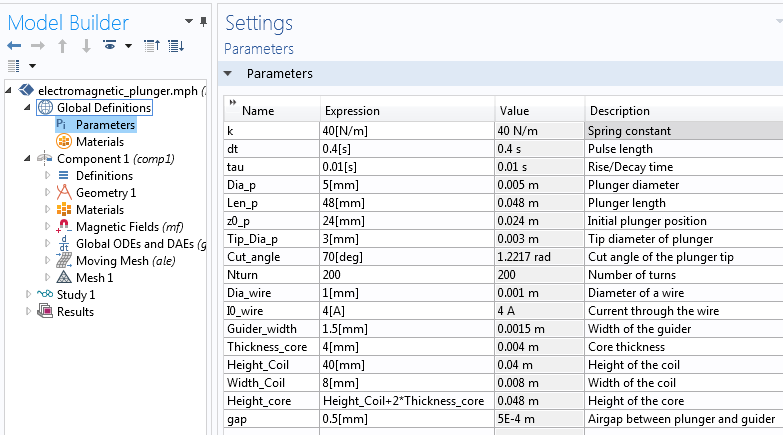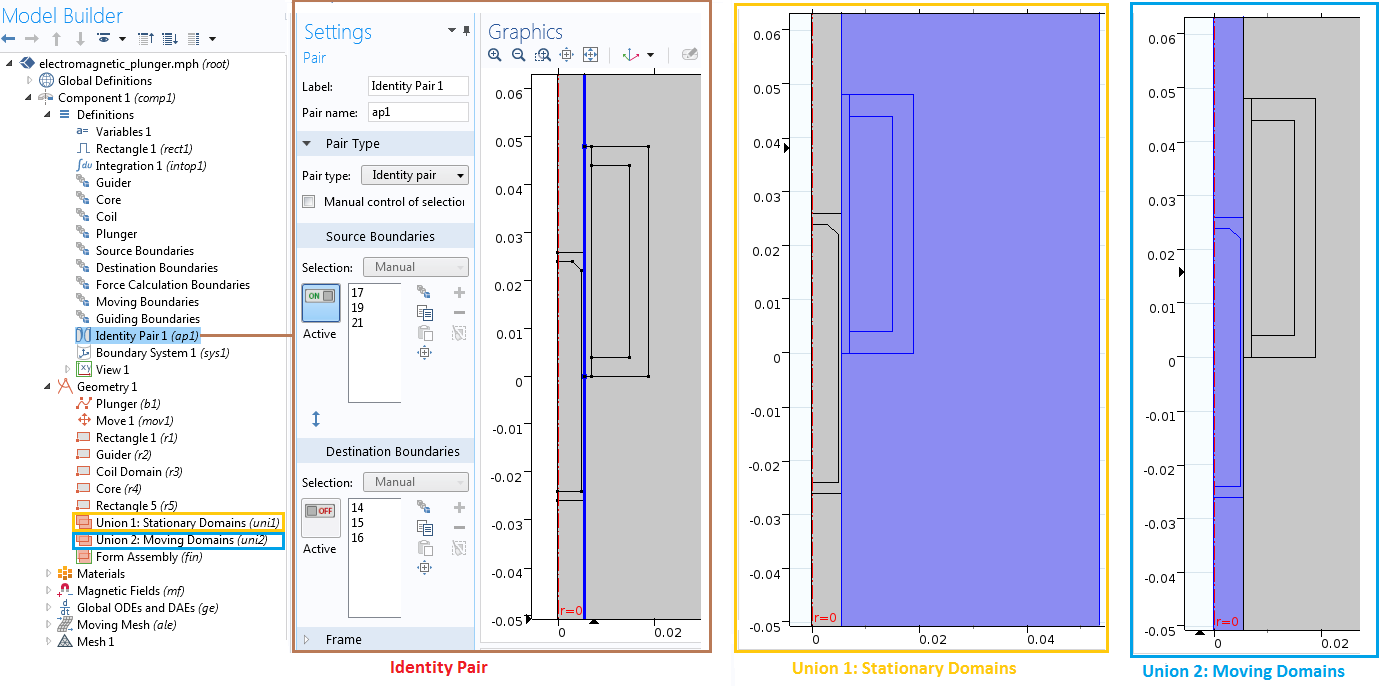“模型开发器”中静态域、移动域和一致对选项。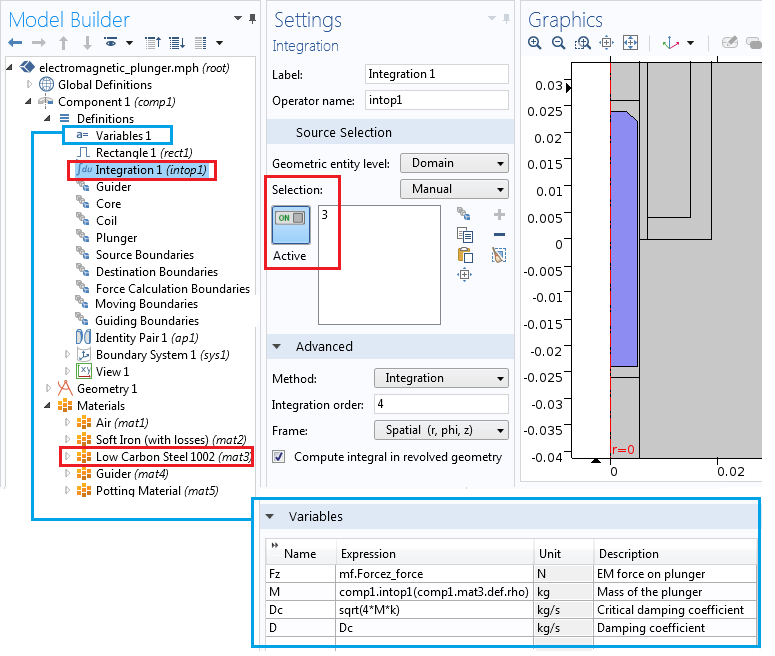模拟电磁场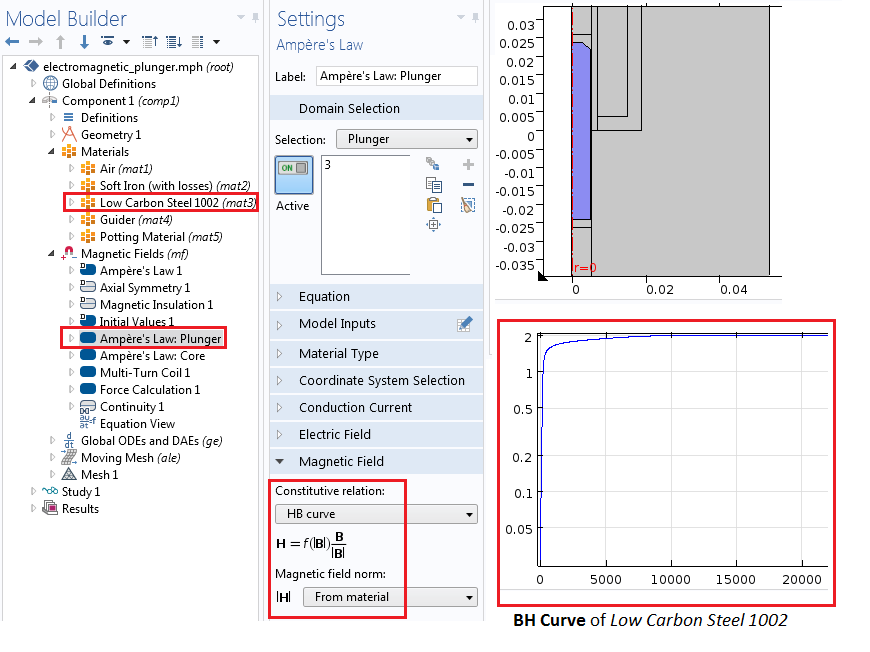“多匝线圈”特征包含了开路、匝数、电导率及横截面积等内容的设置。

添加全局常微分和微分代数方程

(1)

M \frac
{d^2p} {dt^2}
+D\frac
{dp}{dt}+kp-F_z(p,v,t)=0

(2)

M \frac{dv}{dt}+Dv+kp-F_z(p,v,t)=0

(3)

\frac{dp} {dt}
-v=0模拟平移运动